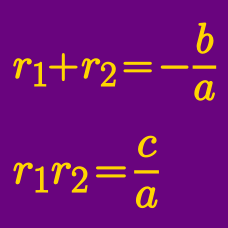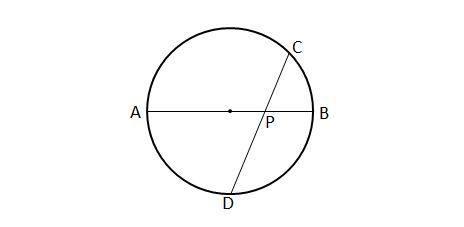Algebra

# Vieta's Formula - Forming Quadratics

Which of the following expresses the quadratic equation whose roots are $p$ and $6$?

A) $x^2 - 6p x + (6 + p) = 0$
B) $x^2 + 6p x - (6+b) = 0$
C) $x^2 + (6 + p) x - 6p = 0$
D) $x^2 - (6+p) x + 6p = 0$In the above diagram, $AB$ is a diameter of the circle with radius $21$ and $CD$ intersects $AB$ at point $P.$ Suppose that $\lvert \overline{CP} \rvert =8$ and $\lvert \overline{DP} \rvert=12.$ If the quadratic equation whose two roots are $\lvert \overline{AP} \rvert$ and $\lvert \overline{BP} \rvert$ can be expressed as $x^2+ax+b=0,$ what is the value of $a+b?$

Which of the following expresses the quadratic equation whose roots are $4$ and $-6$?

A) $x^2 + 2 x + 24 = 0$
B) $x^2 - 2 x + 24 = 0$
C) $x^2 + 2 x - 24 = 0$
D) $x^2 - 2 x - 24 = 0$

Let $\alpha$ and $\beta$ be the two roots of the quadratic equation $x^2-mx+n=0,$ and $\alpha+\beta$ and $\alpha\beta$ be the two roots of the quadratic equation $x^2-8x+1=0.$ Then what is the value of $m^3+n^3 ?$

Let $\alpha$ and $\beta$ be the two roots of the quadration $x^2-2x-2=0.$ If the quadratic equation whose two roots are $\alpha^3$ and $\beta^3$ can be expressed as $x^2+ax+b=0,$ what is the value of $3ab?$

×